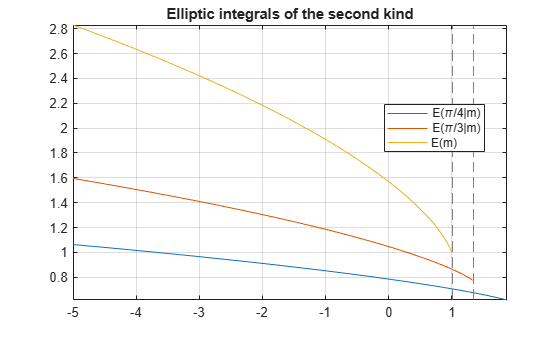ellipticE

Complete and incomplete elliptic integrals of the second kind

Examples

Find Complete Elliptic Integrals of Second Kind

Compute the complete elliptic integrals of the second kind for these numbers. Because these numbers are not symbolic objects, you get floating-point results.

s = [ellipticE(-10.5), ellipticE(-pi/4),...
ellipticE(0),  ellipticE(1)]
s =
3.7096    1.8443    1.5708    1.0000

Compute the complete elliptic integral of the second kind for the same numbers converted to symbolic objects. For most symbolic (exact) numbers, ellipticE returns unresolved symbolic calls.

s = [ellipticE(sym(-10.5)), ellipticE(sym(-pi/4)),...
ellipticE(sym(0)),  ellipticE(sym(1))]
s =
[ ellipticE(-21/2), ellipticE(-pi/4), pi/2, 1]

Use vpa to approximate this result with floating-point numbers:

vpa(s, 10)
ans =
[ 3.70961391, 1.844349247, 1.570796327, 1.0]

Differentiate Elliptic Integrals of Second Kind

Differentiate these expressions involving elliptic integrals of the second kind. ellipticK and ellipticF represent the complete and incomplete elliptic integrals of the first kind, respectively.

syms m
diff(ellipticE(pi/3, m))
diff(ellipticE(m^2), m, 2)
ans =
ellipticE(pi/3, m)/(2*m) - ellipticF(pi/3, m)/(2*m)

ans =
2*m*((ellipticE(m^2)/(2*m^2) -...
ellipticK(m^2)/(2*m^2))/m - ellipticE(m^2)/m^3 +...
ellipticK(m^2)/m^3 + (ellipticK(m^2)/m +...
ellipticE(m^2)/(m*(m^2 - 1)))/(2*m^2)) +...
ellipticE(m^2)/m^2 - ellipticK(m^2)/m^2

Elliptic Integral for Matrix Input

Call ellipticE for this symbolic matrix. When the input argument is a matrix, ellipticE computes the complete elliptic integral of the second kind for each element.

ellipticE(sym([1/3 1; 1/2 0]))
ans =
[ ellipticE(1/3),    1]
[ ellipticE(1/2), pi/2]

Plot Complete and Incomplete Elliptic Integrals of Second Kind

Plot the incomplete elliptic integrals ellipticE(phi,m) for phi = pi/4 and phi = pi/3. Also plot the complete elliptic integral ellipticE(m).

syms m
fplot([ellipticE(pi/4,m) ellipticE(pi/3,m) ellipticE(m)])

title('Elliptic integrals of the second kind')
legend('E(\pi/4|m)','E(\pi/3|m)','E(m)','Location','Best')
grid onInput Arguments

collapse all

Input, specified as a number, vector, matrix, or array, or a symbolic number, variable, array, function, or expression.

Input, specified as a number, vector, matrix, or array, or a symbolic number, variable, array, function, or expression.

collapse all

Incomplete Elliptic Integral of the Second Kind

The incomplete elliptic integral of the second kind is defined as follows:

$E\left(\phi |m\right)=\underset{0}{\overset{\phi }{\int }}\sqrt{1-m{\mathrm{sin}}^{2}\theta }d\theta$

Note that some definitions use the elliptical modulus k or the modular angle α instead of the parameter m. They are related as m = k2 = sin2α.

Complete Elliptic Integral of the Second Kind

The complete elliptic integral of the second kind is defined as follows:

$E\left(m\right)=E\left(\frac{\pi }{2}|m\right)=\underset{0}{\overset{\pi /2}{\int }}\sqrt{1-m{\mathrm{sin}}^{2}\theta }d\theta$

Note that some definitions use the elliptical modulus k or the modular angle α instead of the parameter m. They are related as m = k2 = sin2α.

Tips

• ellipticE returns floating-point results for numeric arguments that are not symbolic objects.

• For most symbolic (exact) numbers, ellipticE returns unresolved symbolic calls. You can approximate such results with floating-point numbers using vpa.

• If m is a vector or a matrix, then ellipticE(m) returns the complete elliptic integral of the second kind, evaluated for each element of m.

• At least one input argument must be a scalar or both arguments must be vectors or matrices of the same size. If one input argument is a scalar and the other one is a vector or a matrix, then ellipticE expands the scalar into a vector or matrix of the same size as the other argument with all elements equal to that scalar.

• ellipticE(pi/2, m) = ellipticE(m).

Alternatives

You can use ellipke to compute elliptic integrals of the first and second kinds in one function call.

 Milne-Thomson, L. M. “Elliptic Integrals.” Handbook of Mathematical Functions with Formulas, Graphs, and Mathematical Tables. (M. Abramowitz and I. A. Stegun, eds.). New York: Dover, 1972.

Symbolic Math Toolbox Documentation

Mathematical Modeling with Symbolic Math Toolbox

Get examples and videos